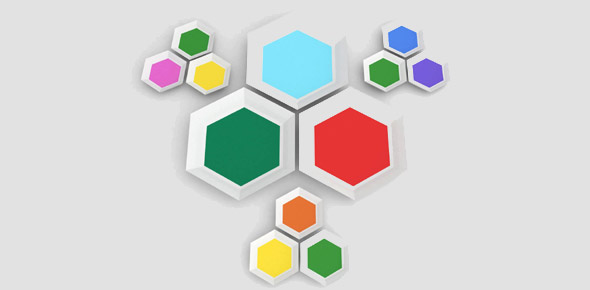# 8 Maths - Perimeter, Area And Volume

12 Questions | Total Attempts: 147SettingsAttempt all questions. Calculators are allowed. Make sure you have working out paper ready (not collected)

• 1.
Name the line that passes through the middle of a circle from one side to the other
• A.

• B.

Chord

• C.

Diameter

• D.

Sector

• 2.
Name this shape.
• A.

Sector

• B.

Semi-circle

• C.

Sphere

• 3.
Circumference =    Find the circumference of the circle above. (14cm diameter)
• A.

44cm

• B.

42cm

• C.

56.21cm

• 4.
Find the area of this triangle. Use the formula
• A.

25cm squared

• B.

12.5cm squared

• C.

15cm squared

• 5.
Find the perimeter. (Answer with a number not a word)
• 6.
Find the area of the above shape. Answer as a whole number only without the cm or squared indice. (A number not a word!)
• 7.
Find the area of the large circle. The 2 smaller circles are the same size. Answer to the nearest whole number... without cm or square indice (A number not a word!)
• 8.
Find the volume of this square prism. 3 x 3 cm base and 19cm long
• A.

171 cubic cm

• B.

191 cubic cm

• C.

81 cubic cm

• 9.
Find the surface area of this triangular prism
• A.

173.1 cm squared

• B.

73.3 cm squared

• C.

28 cm squared x 2

• 10.
Find the volume of the triangular prism. Answer to the nearest whole number without cm or indice. (Write a number not a word!)
• 11.
What 3D object can be made with this net?  (Correct spelling please. don't use capital letters to start the words)
• 12.
This diagram shows 3 balls packed in a cylindrical container. The diameter of each ball is 12 cm. Calculate the volume of the container. (answer to the nearest whole number without cm or indice)
Related TopicsBack to top